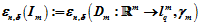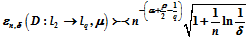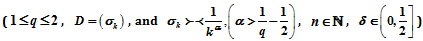# 概率框架下对角算子的熵数Entropy Number of Diagonal Operators under Probabilistic Framework

DOI: 10.12677/AAM.2021.104105, PDF, HTML, XML, 下载: 16  浏览: 39

Abstract: In this paper, we mainly talked about the entropy numbers of the finite dimensional diagonal operatorswhich satisfied, and infinite dimensional diagonal operatorswhich satisfiedand estimated its asymptotic order.

1. 引言及主要结果

${\epsilon }_{n,\delta }\left(W,\mu ,X\right)=\underset{G}{\mathrm{inf}}{\epsilon }_{n}\left(W\G,X\right)$

${\epsilon }_{n,\delta }\left(T:X\to Y,\mu \right)=\underset{G\in B}{\mathrm{inf}}{\epsilon }_{n}\left(T\left(X\G\right),Y\right)$

${\gamma }_{m}\left(G\right)={\left(2\pi \right)}^{-\frac{m}{2}}{\int }_{G}\mathrm{exp}\left(-\frac{1}{2}{‖x‖}_{{l}_{2}^{m}}^{2}\right)\text{d}x\text{ }\text{ }.$

G是 ${ℝ}^{m}$ 中任意一个Borel可测集，易见 ${\gamma }_{m}\left({ℝ}^{m}\right)=1$。那么， ${ℝ}^{m}$${l}_{q}^{m}$ 空间中关于标准高斯测度 ${\gamma }_{m}$ 的熵数就可表示为：

${\epsilon }_{n,\delta }\left({ℝ}^{m},{\gamma }_{m},{l}_{q}^{m}\right)=\underset{G}{\mathrm{inf}}{\epsilon }_{n}\left({ℝ}^{m}\G,{l}_{q}^{m}\right),$

G是跑遍所有满足 ${\gamma }_{m}\left(G\right)\le \delta$ 的Borel子集。

${e}_{n}\left(n\in \stackrel{0}{ℕ}=ℕ-\left\{0\right\}\right)$ 对应的特征值为 ${\lambda }_{n}={n}^{-\rho }$ $\left(\rho >1\right)$，即

${C}_{\mu }{e}_{n}={\lambda }_{n}{e}_{n},n\in \stackrel{0}{ℕ}$

${y}_{1},\cdots ,{y}_{n}$${l}_{2}$ 中任一正交系， ${\xi }_{j}=〈{C}_{\mu }{y}_{j},{y}_{j}〉$$j=1,\cdots ,n$，B为 ${ℝ}^{n}$ 中任一Borel集，则 ${l}_{2}$ 中柱集

$G=\left\{x\in {l}_{2}|\text{\hspace{0.17em}}\left(〈x,{y}_{1}〉,\cdots ,〈x,{y}_{n}〉\right)\in B\right\}$

$\mu \left(G\right)=\underset{j=1}{\overset{n}{\prod }}{\left(2\pi {\xi }_{j}\right)}^{-\frac{1}{2}}{\int }_{B}\mathrm{exp}\left(-\underset{j=1}{\overset{n}{\sum }}\frac{{|{\mu }_{j}|}^{2}}{2{\xi }_{j}}\right)\text{d}{\mu }_{1}\cdots \text{d}{\mu }_{n}.$

${S}_{k}=\left\{n\in \stackrel{0}{ℕ}|{2}^{k-1}\le n<{2}^{k}\right\}.$

${F}_{k}=span\left\{{e}_{n}|n\in {S}_{k}\right\}.$

${I}_{k}:{F}_{k}\to {ℝ}^{{m}_{k}}$

$x=\underset{n\in {S}_{k}}{\sum }{x}_{n}{e}_{n}↦{I}_{k}x=\underset{j=1}{\overset{{m}_{k}}{\sum }}{x}_{{2}^{k-1}+j-1}{{e}^{\prime }}_{j},$

${I}_{k}$${F}_{k}$${ℝ}^{{m}_{k}}$ 上的线性同构，且

${‖x‖}_{{l}_{q}}={‖{I}_{k}x‖}_{{l}_{q}^{{m}_{{}_{k}}}}={‖{\left\{〈x,{e}_{{2}^{k-1}+j-1}〉\right\}}_{j=1}^{{m}_{k}}‖}_{{l}_{q}^{{m}_{{}_{k}}}}\left(1\le p\le \infty \right).$

${\xi }_{n}=〈{C}_{\mu }{e}_{n},{e}_{n}〉={n}^{-\rho },$

$\frac{1}{{2}^{k\rho }}<{\xi }_{n}\le \frac{{2}^{\rho }}{{2}^{k\rho }}.$

${{\xi }^{\prime }}_{k}=\frac{1}{{2}^{k\rho }}$${{\xi }^{″}}_{k}=\frac{{2}^{\rho }}{{2}^{k\rho }}$${\stackrel{¯}{\xi }}_{k}=\left({\xi }_{{2}^{k-1}},\cdots ,{\xi }_{{2}^{k}-1}\right)$

$x=\left({x}_{1},\cdots ,{x}_{m}\right),y=\left({y}_{1},\cdots ,{y}_{m}\right),\beta \in ℝ,M\subset {ℝ}^{m}$$1\le q\le \infty$，记

$xy=\left({x}_{1}{y}_{1},\cdots ,{x}_{m}{y}_{m}\right),{x}^{\beta }=\left({x}_{1}^{\beta },\cdots ,{x}_{m}^{\beta }\right),e\left(x,M,{l}_{q}^{m}\right)=\underset{y\in M}{\mathrm{inf}}{‖x-y‖}_{{l}_{q}^{m}}.$

2. 主要结果的证明

${\epsilon }_{n,\delta }\left({I}_{m}\right):={\epsilon }_{n,\delta }\left({I}_{m}:{ℝ}^{m}\to {l}_{q}^{m},{\gamma }_{m}\right)={\epsilon }_{n,\delta }\left({ℝ}^{m},{l}_{q}^{m},{\gamma }_{m}\right)\succ \prec {2}^{-\frac{n}{m}}{m}^{\frac{1}{q}-\frac{1}{2}}\sqrt{m+\mathrm{ln}\frac{1}{\delta }}\text{\hspace{0.17em}}.$

$\underset{1\le k\le m}{\mathrm{sup}}{2}^{-\frac{n}{k}}{\left({\sigma }_{1}\cdots {\sigma }_{k}\right)}^{\frac{1}{k}}{k}^{\frac{1}{q}-\frac{1}{p}}\ll {\epsilon }_{n}\left({D}_{m}\right)\ll \underset{1\le k\le m}{\mathrm{sup}}{2}^{-\frac{n}{k}}{\left({\sigma }_{1}\cdots {\sigma }_{k}\right)}^{\frac{1}{k}}{m}^{\frac{1}{q}-\frac{1}{p}}.$

$\underset{1\le k\le m}{\mathrm{sup}}{2}^{-\frac{n}{k}}{\left({\sigma }_{1}\cdots {\sigma }_{k}\right)}^{\frac{1}{k}}\cdot {k}^{\frac{1}{q}-\frac{1}{2}}\sqrt{k+\mathrm{ln}\frac{1}{\delta }}\ll {\epsilon }_{n,\delta }\left({D}_{m}:{ℝ}^{m}\to {l}_{q}^{m},{\gamma }_{m}\right)\ll \underset{1\le k\le m}{\mathrm{sup}}{2}^{-\frac{n}{k}}{\left({\sigma }_{1}\cdots {\sigma }_{k}\right)}^{\frac{1}{k}}\cdot {m}^{\frac{1}{q}-\frac{1}{2}}\sqrt{m+\mathrm{ln}\frac{1}{\delta }}\text{\hspace{0.17em}}.$

${\gamma }_{m}\left(x\in {ℝ}^{m}{|\text{ }‖x‖}_{{l}_{2}^{m}}>{C}_{0}\sqrt{m+\mathrm{ln}\frac{1}{\delta }}\right)\le \delta .$

$\begin{array}{c}{\epsilon }_{n,\delta }\left({D}_{m}:{ℝ}^{m}\to {l}_{q}^{m},{\gamma }_{m}\right)\le {\epsilon }_{n}\left({D}_{m}\left({C}_{0}\sqrt{m+\mathrm{ln}\frac{1}{\delta }}\right){B}_{2}^{m},{l}_{q}^{m}\right)\\ ={C}_{0}\sqrt{m+\mathrm{ln}\frac{1}{\delta }}{\epsilon }_{n}\left({D}_{m}\left({B}_{2}^{m}\right),{l}_{q}^{m}\right)\\ \ll \underset{1\le k\le m}{\mathrm{sup}}{2}^{-\frac{n}{k}}{\left({\sigma }_{1}\cdots {\sigma }_{k}\right)}^{\frac{1}{k}}\cdot {m}^{\frac{1}{q}-\frac{1}{2}}\sqrt{m+\mathrm{ln}\frac{1}{\delta }}\text{\hspace{0.17em}}.\end{array}$

$1\le k\le m$

${I}_{k}:{l}_{2}^{k}\to {l}_{2}^{m}$

$\left({x}_{1},\cdots ,{x}_{k}\right)↦\left({x}_{1},\cdots ,{x}_{k},0,\cdots ,0\right),$

${P}_{k}:{l}_{q}^{m}\to {l}_{q}^{k}$

$\left({x}_{1},\cdots ,{x}_{k},\cdots ,{x}_{m}\right)↦\left({x}_{1},\cdots ,{x}_{k}\right),$

${D}_{k}:{l}_{2}^{k}\to {l}_{q}^{k}$，则 ${D}_{k}={P}_{k}{D}_{m}{I}_{k}$

${\epsilon }_{n,\delta }\left({D}_{m}:{ℝ}^{m}\to {l}_{q}^{m},{\gamma }_{m}\right)={\epsilon }_{n}\left({D}_{m}\left({Q}_{m}\right),{l}_{q}^{m}\right).$

${D}_{m}\left({Q}_{m}\right)\subset \underset{j=1}{\overset{l}{\cup }}\left\{{y}_{j}+{\epsilon }_{n,\delta }{B}_{q}^{m}\right\}\text{ }\text{ }.$

${Q}_{k}={P}_{k}{Q}_{m}$，则 ${\gamma }_{k}\left({Q}_{k}\right)\ge 1-\delta$。若不然， ${\gamma }_{k}\left({Q}_{k}\right)<1-\delta$，令

$F=\left\{\left({x}_{1},\cdots ,{x}_{k},{x}_{k+1},\cdots ,{x}_{m}\right)|\left({x}_{1},\cdots ,{x}_{k}\right)\in {Q}_{k},-\infty <{x}_{j}<\infty ,j=k+1,\cdots ,m\right\},$

${\gamma }_{m}\left(F\right)={\gamma }_{k}\left({Q}_{k}\right)<1-\delta$，而 ${Q}_{m}\subset F$，矛盾。

${\epsilon }_{n,\delta }\left({D}_{k}:{ℝ}^{k}\to {l}_{q}^{k},{\gamma }_{k}\right)\le {\epsilon }_{n,\delta }\left({D}_{k}\left({Q}_{k}\right),{l}_{q}^{k},{\gamma }_{k}\right).$

${D}_{k}\left({Q}_{k}\right)\subset \underset{j=1}{\overset{l}{\cup }}\left\{{P}_{k}{y}_{j}+{\epsilon }_{n,\delta }{B}_{q}^{k}\right\}\text{ }\text{ }.$

${\epsilon }_{n,\delta }\left({D}_{k}:{ℝ}^{k}\to {l}_{q}^{k},{\gamma }_{k}\right)\ll {\epsilon }_{n,\delta }\left({D}_{m}:{ℝ}^{m}\to {l}_{q}^{m},{\gamma }_{m}\right).$

$D=G\cap {G}_{{t}_{1}},{D}_{1}=G-D,{D}_{2}={G}_{{t}_{1}}-D$。则

$\left\{\begin{array}{l}{‖x‖}_{{l}_{2}^{k}}\le {t}_{1}\text{ }x\in {D}_{1}\\ {‖x‖}_{{l}_{2}^{k}}\ge {t}_{1}\text{ }x\in {D}_{2}\end{array}$

$Vo{l}_{k}\left({ℝ}^{k}-G\right)\ge Vo{l}_{k}\left({ℝ}^{k}-{G}_{{t}_{1}}\right),$

$\begin{array}{c}{2}^{\frac{n}{k}}{\epsilon }_{n}\left({D}_{k}\left({ℝ}^{k}-{G}_{{t}_{1}}\right),{l}_{q}^{k}\right)\ge {\left(\frac{Vo{l}_{k}\left({D}_{k}\left({ℝ}^{k}-{G}_{{t}_{1}}\right)\right)}{Vo{l}_{k}\left({B}_{q}^{k}\right)}\right)}^{\frac{1}{k}}\\ \ge {\left({\sigma }_{1}\cdots {\sigma }_{k}\right)}^{\frac{1}{k}}{\left(\frac{Vo{l}_{k}\left(\left({ℝ}^{k}-{G}_{{t}_{1}}\right)\right)}{Vo{l}_{k}\left({B}_{q}^{k}\right)}\right)}^{\frac{1}{k}}\\ \ge {\left({\sigma }_{1}\cdots {\sigma }_{k}\right)}^{\frac{1}{k}}{\left(\frac{{t}_{1}^{k}Vo{l}_{k}\left({B}_{2}^{k}\right)}{Vo{l}_{k}\left({B}_{q}^{k}\right)}\right)}^{\frac{1}{k}}\\ \ge {\left({\sigma }_{1}\cdots {\sigma }_{k}\right)}^{\frac{1}{k}}{k}^{\frac{1}{q}-\frac{1}{2}}\sqrt{k+\mathrm{ln}\frac{1}{\delta }}\text{ }\text{ }.\end{array}$

${\epsilon }_{n}\left({D}_{k}\left({ℝ}^{k}-G\right),{l}_{q}^{k}\right)\ge {2}^{-\frac{n}{k}}{\left({\sigma }_{1}\cdots {\sigma }_{k}\right)}^{\frac{1}{k}}{k}^{\frac{1}{q}-\frac{1}{2}}\sqrt{k+\mathrm{ln}\frac{1}{\delta }},$

${\epsilon }_{n,\delta }\left({D}_{k}:{ℝ}^{k}\to {l}_{q}^{k},{\gamma }_{k}\right)\gg {2}^{-\frac{n}{k}}{\left({\sigma }_{1}\cdots {\sigma }_{k}\right)}^{\frac{1}{k}}{k}^{\frac{1}{q}-\frac{1}{2}}\sqrt{k+\mathrm{ln}\frac{1}{\delta }},$

${\epsilon }_{n,\delta }\left({D}_{m}:{ℝ}^{m}\to {l}_{q}^{m},{\gamma }_{m}\right)\gg \underset{1\le k\le m}{\mathrm{sup}}{2}^{-\frac{n}{k}}{\left({\sigma }_{1}\cdots {\sigma }_{k}\right)}^{\frac{1}{k}}{k}^{\frac{1}{q}-\frac{1}{2}}\sqrt{k+\mathrm{ln}\frac{1}{\delta }}.$

${\sigma }_{1}\ge {\sigma }_{2}\ge \cdots \ge {\sigma }_{k}\ge \cdots >0,n\in ℕ,\delta \in \left(0,\frac{1}{2}\right],n\in \stackrel{0}{ℕ}$

$\left\{{n}_{k}\right\}$ 为非负整数列， $\left\{{\delta }_{k}\right\}$ 为非负数列，且满足

$\underset{k=1}{\overset{\infty }{\sum }}{n}_{k}\le n,\text{ }\underset{k=1}{\overset{\infty }{\sum }}{\delta }_{k}\le \delta .$

${\epsilon }_{n,\delta }\left(D\right):={\epsilon }_{n}\left(D:{l}_{2}\to {l}_{q},\mu \right)\ll \underset{k=1}{\overset{\infty }{\sum }}{2}^{-\frac{k}{2}\rho }{\epsilon }_{{n}_{k},{\delta }_{k}}\left({D}_{k}:{ℝ}^{{m}_{k}}\to {l}_{q}^{{m}_{k}},{\gamma }_{{m}_{k}}\right).$

${D}_{k}:{l}_{2}^{{m}_{k}}\to {l}_{q}^{{m}_{k}}$

$x=\left({x}_{1},\cdots ,{x}_{{m}_{k}}\right)↦{D}_{k}x=\left({\sigma }_{{2}^{k-1}}{x}_{1},\cdots ,{\sigma }_{{2}^{k}-1}{x}_{{2}^{k}-1}\right).$

${\gamma }_{{m}_{k}}\left(\left\{y\in {ℝ}^{{m}_{k}}|e\left({D}_{k}y,{M}_{k},{l}_{q}^{{m}_{k}}\right)>{\epsilon }_{{n}_{k},{\delta }_{k}}\right\}\right)\le {\delta }_{k}.$

${D}^{k}:{l}_{2}\to {l}_{q}$

$x=\left({x}_{n}\right)↦{D}^{k}x=\left(0,\cdots ,0,\cdots ,{\sigma }_{{2}^{k-1}}{x}_{{2}^{k-1}},\cdots ,{\sigma }_{{2}^{k}-1}{x}_{{2}^{k}-1},0,\cdots \right),$

$x\in {l}_{2}$，则 ${D}^{k}x\in {F}_{k}$。易见

$e\left({D}^{k}x,{I}_{k}^{-1}{M}_{k},{l}_{q}\right)=e\left({\left\{〈{D}^{k}x,{e}_{{2}^{k-1}+j-1}〉\right\}}_{j=1}^{{m}_{k}},{M}_{k},{l}_{q}^{{m}_{k}}\right).$

${G}_{k}=\left\{x\in {l}_{2}|e\left({D}^{k}x,{I}_{k}^{-1}{M}_{k},{l}_{q}\right)>{{\xi }^{″}}_{k}{}^{\text{\hspace{0.17em}}\frac{1}{2}}{\epsilon }_{{n}_{k},{\delta }_{k}}\right\}.$

$\begin{array}{c}\mu \left({G}_{k}\right)=\mu \left(\left\{x\in {l}_{2}|e\left({\left\{〈{D}^{k}x,{e}_{{2}^{k-1}+j-1}〉\right\}}_{j=1}^{{m}_{k}},{M}_{k},{l}_{q}^{{m}_{k}}\right)\ge {{\xi }^{″}}_{k}^{\frac{1}{2}}{\epsilon }_{{n}_{k},{\delta }_{k}}\right\}\right)\\ ={\gamma }_{{m}_{k}}\left(\left\{y\in {ℝ}^{{m}_{k}}|e\left(e\left({D}_{k}y\right){\stackrel{¯}{\xi }}_{k}^{\frac{1}{2}},{M}_{k}^{}{\stackrel{¯}{\xi }}^{\frac{1}{2}},{l}_{q}^{{m}_{k}}\right)>{{\xi }^{″}}_{k}^{\frac{1}{2}}{\epsilon }_{{n}_{k},{\delta }_{k}}\right\}\right)\\ \le {\gamma }_{{m}_{k}}\left(\left\{y\in {ℝ}^{{m}_{k}}|{{\xi }^{″}}_{k}^{\frac{1}{2}}e\left({D}_{k}y,{M}_{k},{l}_{q}^{{m}_{k}}\right)>{{\xi }^{″}}_{k}^{\frac{1}{2}}{\epsilon }_{{n}_{k},{\delta }_{k}}\right\}\right)\\ ={\gamma }_{{m}_{k}}\left(\left\{y\in {ℝ}^{{m}_{k}}|e\left({D}_{k}y,{M}_{k},{l}_{q}^{{m}_{k}}\right)>{\epsilon }_{{n}_{k},{\delta }_{k}}\right\}\right)\\ \le {\delta }_{k}.\end{array}$

$G=\underset{k=1}{\overset{\infty }{\cup }}{G}_{k},M=\sum {I}_{k}^{-1}{M}_{k}$

$\mu \left(G\right)\le \sum \mu \left({G}_{n}\right)\le \sum {\delta }_{k}\le \delta ,$

$|M|\le {2}^{\underset{n=1}{\overset{\infty }{\sum }}{n}_{k}}\le {2}^{n}.$

$\begin{array}{c}{\epsilon }_{n,\delta }\left(D:{l}_{2}\to {l}_{q},\mu \right)\ll \underset{x\in {l}_{2}\G}{\mathrm{sup}}e\left(Dx,M,{l}_{q}\right)\\ \ll \underset{x\in {l}_{2}\G}{\mathrm{sup}}\sum e\left({D}_{k}x,{I}_{k}^{-1}{M}_{k},{l}_{q}\right)\\ \ll \underset{k}{\sum }{2}^{-\frac{k}{2}\rho }{\epsilon }_{{n}_{k},{\delta }_{k}}\left({D}_{k}:{ℝ}^{{m}_{k}}\to {l}_{q}^{{m}_{k}},{\gamma }_{{m}_{k}}\right).\end{array}$

${\epsilon }_{n,\delta }\left(D:{l}_{2}\to {l}_{q},\mu \right)\gg {2}^{-\frac{k}{2}\rho }{\epsilon }_{n,\delta }\left({D}_{k}:{ℝ}^{{m}_{k}}\to {l}_{q}^{{m}_{k}},{\gamma }_{{m}_{k}}\right),$

${D}_{k}:{l}_{2}^{{m}_{k}}\to {l}_{q}^{{m}_{k}}$

$x=\left({x}_{1},\cdots ,{x}_{{m}_{k}}\right)↦{D}_{k}x=\left({\sigma }_{{2}^{k-1}}{x}_{1},\cdots ,{\sigma }_{{2}^{k}-1}{x}_{{2}^{k}-1}\right).$

$\forall j\in S$${\xi }_{j}=〈{C}_{\mu }{e}_{j},{e}_{j}〉={j}^{-\rho }$，则 $\frac{1}{{2}^{\rho k}{2}^{\rho }}<{\xi }_{j}\le \frac{{2}^{\rho }}{{2}^{\rho k}}$。令

${{\xi }^{\prime }}_{k}=\frac{1}{{2}^{\rho k}},{{\xi }^{″}}_{k}=\frac{{2}^{\rho }}{{2}^{\rho k}},{\stackrel{¯}{\xi }}_{k}=\left({\xi }_{{2}^{k-1}},\cdots ,{\xi }_{{2}^{k}-1}\right).$

${M}_{1}$${l}_{q}\cap {F}_{s}$ 中的子集，且 $|M|\le {2}^{n}$，则

$\mu \left\{x\in {l}_{2}\cap {F}_{s}|e\left({D}_{k}x,{M}_{1},{l}_{q}\cap {F}_{s}\right)>{\epsilon }_{n,\delta }\right\}\le \delta .$

${I}_{s}:{F}_{s}\to {ℝ}^{{m}_{k}}$

$x=\underset{j\in s}{\sum }{x}_{j}{e}_{j}↦{I}_{s}x=\underset{j=1}{\overset{{m}_{k}}{\sum }}{x}_{{2}^{k-1}+j-1}{{e}^{\prime }}_{j}.$

${I}_{s}$${F}_{s}$${ℝ}^{{m}_{k}}$ 线性算子，且

${‖x‖}_{{l}_{q}}=‖{I}_{s}x‖={‖{\left\{〈x,{e}_{{2}^{k-1}+j-1}〉\right\}}_{j=1}^{{m}_{k}}‖}_{{l}_{q}^{m}}.$

$G=\left\{y\in {ℝ}^{{m}_{k}}|e\left\{\left({D}_{k}y\right),\left({I}_{s}{M}_{1}\right),{l}_{q}^{{m}_{k}}\right\}>{{\xi }^{\prime }}_{k}^{{}^{-\frac{1}{2}}}{\epsilon }_{n,\delta }\right\}.$

$\begin{array}{c}{\gamma }_{{m}_{k}}\left(G\right)={\gamma }_{{m}_{k}}\left(\left\{y\in {ℝ}^{{m}_{k}}|e\left(\left({D}_{k}y\right){{\xi }^{\prime }}_{k}^{{}^{\frac{1}{2}}},\left({I}_{s}{M}_{1}\right){{\xi }^{\prime }}_{k}^{{}^{\frac{1}{2}}},{l}_{q}^{{m}_{k}}\right)>{\epsilon }_{n,\delta }\right\}\right)\\ \le {\gamma }_{{m}_{k}}\left(\left\{y\in {ℝ}^{{m}_{k}}|e\left(\left({D}_{k}y\right){\stackrel{¯}{\xi }}_{k}^{{}^{\frac{1}{2}}},\left({I}_{s}{M}_{1}\right){\stackrel{¯}{\xi }}_{k}^{{}^{\frac{1}{2}}},{l}_{q}^{{m}_{k}}\right)>{\epsilon }_{n,\delta }\right\}\right)\\ =\mu \left(\left\{x\in {l}_{2}\cap {F}_{s}|e\left({\left(Dx,{e}_{j}\right)}_{j\in s},{I}_{s}{M}_{1},{l}_{q}^{{m}_{k}}\right)>{\epsilon }_{n,\delta }\right\}\right)\\ \le \mu \left(\left\{x\in {l}_{2}\cap {F}_{s}|e\left(Dx,{M}_{1},{l}_{q}\right)>{\epsilon }_{n,\delta }\right\}\right)<\delta .\end{array}$

$\begin{array}{c}{\epsilon }_{n,\delta }\left({D}_{k}:{ℝ}^{{m}_{k}}\to {l}_{q}^{{m}_{k}},{\gamma }_{{m}_{k}}\right)\le e\left({D}_{k}\left({ℝ}^{{m}_{k}}\G\right),{I}_{s}{M}_{1},{l}_{q}^{{m}_{k}}\right)\\ =\underset{y\in {ℝ}^{{m}_{k}}\G}{\mathrm{sup}}e\left({D}_{k}y,{I}_{s}{M}_{1},{l}_{q}^{{m}_{k}}\right)\\ \ll {2}^{\frac{\rho }{2}k}{\epsilon }_{n,\delta }.\end{array}$

${\epsilon }_{n,\delta }\left(D:{l}_{2}\to {l}_{q},\mu \right)\gg {2}^{-\frac{\rho }{2}k}{\epsilon }_{n,\delta }\left({D}_{k}:{ℝ}^{{m}_{k}}\to {l}_{q}^{{m}_{k}},{\gamma }_{{m}_{k}}\right).$

${\epsilon }_{n,\delta }\left(D:{l}_{2}\to {l}_{q},\mu \right)\succ \prec {n}^{-\left(\alpha +\frac{\rho }{2}-\frac{1}{q}\right)}\sqrt{1+\frac{1}{n}\mathrm{ln}\frac{1}{\delta }}\text{ }\text{ }.$

$\forall k\in ℕ$，令

${n}_{k}=\left\{\begin{array}{l}{2}^{k}{2}^{\left(1-\beta \right)\left({k}^{\prime }-k\right)},k\le {k}^{\prime }\\ {2}^{k}{2}^{\left(1+\beta \right)\left({k}^{\prime }-k\right)},k>{k}^{\prime }\end{array},{\delta }_{k}=\frac{\delta \cdot {n}_{k}}{n},$

$\sum {n}_{k}\le n,\sum {\delta }_{k}\le \delta$，且由引理2.1及定理2.5，得

$\begin{array}{c}{\epsilon }_{n,\delta }\left(D:{l}_{2}\to {l}_{q},\mu \right)\ll \underset{k=1}{\overset{\infty }{\sum }}{2}^{-\frac{\rho k}{2}}{\epsilon }_{{n}_{k},{\delta }_{k}}\left({D}_{k}:{ℝ}^{{m}_{k}}\to {l}_{q}^{{m}_{k}},{\gamma }_{{m}_{k}}\right)\\ =\underset{k\le {k}^{\prime }}{\sum }{2}^{-\frac{\rho k}{2}}{\epsilon }_{{n}_{k},{\delta }_{k}}\left({D}_{k}:{ℝ}^{{m}_{k}}\to {l}_{q}^{{m}_{k}},{\gamma }_{{m}_{k}}\right)+\underset{k>{k}^{\prime }}{\sum }{2}^{-\frac{\rho k}{2}}{\epsilon }_{{n}_{k},{\delta }_{k}}\left({D}_{k}:{ℝ}^{{m}_{k}}\to {l}_{q}^{{m}_{k}},{\gamma }_{{m}_{k}}\right)\\ ={I}_{1}+{I}_{2}.\end{array}$

$\begin{array}{c}{I}_{1}\ll {\underset{k\le {k}^{\prime }}{\sum }{2}^{-\frac{\rho k}{2}}\underset{1\le j\le {m}_{k}}{\mathrm{sup}}{2}^{-\frac{{n}_{k}}{j}}{\epsilon }_{{n}_{k},{\delta }_{k}}\left(\frac{1}{{2}^{\left(k-1\right)\alpha }}\cdots \frac{1}{{\left({2}^{k-1}+j-1\right)}^{\alpha }}\right)}^{\frac{1}{j}}\cdot {m}_{k}^{\frac{1}{q}-\frac{1}{2}}\sqrt{{m}_{k}+\mathrm{ln}\frac{1}{{\delta }_{k}}}\\ \ll \underset{k\le {k}^{\prime }}{\sum }{2}^{-\frac{\rho k}{2}}{2}^{-\frac{{2}^{k}{2}^{\left(1-\beta \right)\left({k}^{\prime }-k\right)}}{{2}^{k-1}}}\cdot \frac{1}{{2}^{k\rho }}\cdot {2}^{\frac{k}{q}}+\underset{k\le {k}^{\prime }}{\sum }{2}^{-\frac{\rho k}{2}}{2}^{-\frac{{2}^{k}{2}^{\left(1-\beta \right)\left({k}^{\prime }-k\right)}}{{2}^{k-1}}}\cdot \frac{1}{{2}^{k\rho }}\cdot {2}^{\left(\frac{1}{q}-\frac{1}{2}\right)k}\sqrt{\mathrm{ln}\frac{1}{\delta }\frac{{2}^{k}}{{2}^{k}{2}^{\left(1-\beta \right)\left({k}^{\prime }-k\right)}}}\\ \ll \underset{k\le {k}^{\prime }}{\sum }{2}^{-\left(\frac{\rho }{2}+\alpha -\frac{1}{q}\right)k}{2}^{-2\cdot {2}^{\left(1-\beta \right)\left({k}^{\prime }-k\right)}}+\underset{k\le {k}^{\prime }}{\sum }{2}^{-\left(\frac{\rho }{2}+\alpha -\frac{1}{q}+\frac{1}{2}\right)k}\cdot {2}^{-2\cdot {2}^{\left(1-\beta \right)\left({k}^{\prime }-k\right)}}\sqrt{\beta \left({k}^{\prime }-k\right)}\\ \ll {2}^{-\left(\frac{\rho }{2}+\alpha -\frac{1}{q}\right){k}^{\prime }}\underset{0\le \xi \le {k}^{\prime }-1}{\sum }{2}^{\left(\frac{\rho }{2}-\frac{1}{q}\right)\xi -{2}^{\left(1-\beta \right)\xi }}+{2}^{-\left(\frac{\rho }{2}+\alpha -\frac{1}{q}+\frac{1}{2}\right){k}^{\prime }}\underset{0\le \xi \le {k}^{\prime }}{\sum }{2}^{\left(\frac{\rho }{2}-\frac{1}{q}+\frac{1}{2}\right)\xi -2\cdot {2}^{\left(1-\beta \right)\xi }}\sqrt{\beta \xi }\sqrt{\text{\hspace{0.17em}}\mathrm{ln}\frac{1}{\delta }}\\ \ll {2}^{-\left(\frac{\rho }{2}+\alpha -\frac{1}{q}\right){k}^{\prime }}+{2}^{-\left(\frac{\rho }{2}+\alpha -\frac{1}{q}+\frac{1}{2}\right){k}^{\prime }}\sqrt{\mathrm{ln}\frac{1}{\delta }}\ll {n}^{-\left(\frac{\rho }{2}+\alpha -\frac{1}{q}\right)}\sqrt{1+\frac{1}{n}\mathrm{ln}\frac{1}{\delta }}.\end{array}$

$\begin{array}{c}{I}_{2}\ll {\underset{k>{k}^{\prime }}{\sum }{2}^{-\frac{\rho k}{2}}\underset{1\le j\le {m}_{k}}{\mathrm{sup}}{2}^{-\frac{{n}_{k}}{j}}\left(\frac{1}{{2}^{\left(k-1\right)\alpha }}\cdots \frac{1}{{\left({2}^{k-1}+j-1\right)}^{\alpha }}\right)}^{j}\cdot {2}^{\left(\frac{1}{q}-\frac{1}{2}\right)k}\sqrt{{2}^{k}+\mathrm{ln}\frac{{2}^{-\beta \left({k}^{\prime }-k\right)}}{\delta }}\\ \ll {2}^{-\left(\frac{\rho }{2}+\alpha -\frac{1}{q}\right){k}^{\prime }}\underset{\xi >0}{\sum }{2}^{-\left(\frac{\rho }{2}+\alpha -\frac{1}{q}\right)\xi -2\cdot {2}^{-\left(1+\beta \right)\xi }}+{2}^{-\left(\frac{\rho }{2}+\alpha -\frac{1}{q}\right){k}^{\prime }}\underset{\xi >0}{\sum }{2}^{-\left(\frac{\rho }{2}+\alpha +\frac{1}{2}-\frac{1}{q}\right)\xi -2\cdot {2}^{\left(1-\beta \right)\xi }}\sqrt{\beta \xi }\sqrt{\text{\hspace{0.17em}}\mathrm{ln}\frac{1}{\delta }}\\ \ll {n}^{-\left(\frac{\rho }{2}+\alpha -\frac{1}{q}\right)}\sqrt{1+\frac{1}{n}\mathrm{ln}\frac{1}{\delta }}.\end{array}$

$\begin{array}{c}{\epsilon }_{n,\delta }\gg {2}^{-\frac{\rho k}{2}}{2}^{-\frac{n}{{2}^{k-1}}}\frac{1}{{\left({2}^{k}-1\right)}^{\alpha }}{\left({2}^{k-1}\right)}^{\frac{1}{q}-\frac{1}{2}}\sqrt{{2}^{k-1}+\mathrm{ln}\frac{1}{\delta }}\\ \gg {2}^{-\left(\frac{\rho }{2}+\alpha -\frac{1}{q}+\frac{1}{2}\right)k}\sqrt{{2}^{k}+\mathrm{ln}\frac{1}{\delta }}\\ \gg {n}^{-\left(\frac{\rho }{2}+\alpha -\frac{1}{q}\right)}\sqrt{1+\frac{1}{n}\mathrm{ln}\frac{1}{\delta }}.\end{array}$

  Oloff, R. (1978) Entropieeigenschaften von Diagonaloperatoren. Mathematische Nachrichten, 86, 157-165. https://doi.org/10.1002/mana.19780860115  Tikhominov, V.M. (1976) Some Questions in Approximation Theory. Izdat.Moskov. Gos. Univ., Moscow.  Makavoz, Y.I. (1972) On a Device for Getting Lower Estimates of the Widths of Sets in Banach Spaces. Mat. Sb., 87, 136-142.  Kashin, B.S. (1977) Widths of Certain Finite-Dimensional Sets and Classes of Smooth Function. Izv.Akad. Nauk SSSR, Ser.MAT., 41, 33-351.  Babenko, K.I. (1960) On the Approximation of Periodic Functions of Several Variables by Trigonometric Polynomials. Dokl. Akad. Nauk.SSSR, 132, 247-253.  Galeev, E.M. (1985) Kolmogorov Widths of Classes of Periodic Functions of Many Variables αpW~ and αpH~ in the Space qL. Izv. Akad. Nauk SSSR Ser. Mat., 49, 916-934.  Temlyakov, V.N. (1986) Approximation of Functions with Bounded Mixed Derivative. Tr. Mat. Inst. Akad. Nauk SSSR, 178, 1-112.  Pietsch, A. (1978) Operator Ideals. Mathematische Monographien, Vol. 16. VEB Deutscher Verlagder Wissenschaften, Berlin.  Carl, B. and Stephani, I. (1990) Entropy, Compactness and the Approximation of Operators. Cambridge Tracts in Mathematics, Vol. 98, Cambridge University Press, Cambridge. https://doi.org/10.1017/CBO9780511897467  Edmunds, D.E. and Triebel, H. (1996) Function Spaces, Entropy Numbers, Differential Operator. Cambridge Tracts in Mathematics, Vol. 120, Cambridge University Press, Cambridge. https://doi.org/10.1017/CBO9780511662201  Lorentz, G. (1985) Manfred v. Golitschek, Yuly Makovoz, Constructive Approximation. Springer, Berlin.  Belinsky, E.S. (1998) Estimates of Entropy Numbers and Gaussian Measures for Classes of Functions with Bounded Mixed Derivative. Journal of Approximation Theory, 93, 114-127. https://doi.org/10.1006/jath.1997.3157  Dung, D. (2001) Non-Linear Approximations Using of Finite Cardinality or Finite Pseudo-Dimension. Journal of Complexity, 17, 467-492. https://doi.org/10.1006/jcom.2001.0579  Schütt, C. (1984) Entropy Numbers of Diagonal Operators between Symmetric Banach Spaces. Journal of Approximation Theory, 40, 121-128. https://doi.org/10.1016/0021-9045(84)90021-2  Carl, B. (1980) Entropy Numbers of Diagonal Operators with an Application to Eigenvalue Problems. Journal of Approximation Theory, 32, 135-150. https://doi.org/10.1016/0021-9045(81)90110-6  Kühn, T. (2005) Entropy Numbers of General Diagonal Operators. Revista Matamatica Complutense, 18, 479-491. https://doi.org/10.5209/rev_REMA.2005.v18.n2.16697  Marcus, M.B. (1974) The ε-Entropy of Some Compact Subsets of . Journal of Approximation Theory, 10, 304-312. https://doi.org/10.1016/0021-9045(74)90102-6  韩永杰. 概率框架和平均框架下的熵数[D]: [硕士学位论文]. 成都: 西华大学, 2011.  王桐心. 不同框架下对角算子的熵数[D]: [硕士学位论文]. 成都: 西华大学, 2018.  Chen, J., Lu, W., Xiao, H., Wang, Y. and Tan, X. (2019) Entropy Number of Diagonal Operator. Journal of Applied Mathematics and Physics, 7, 738-745. https://doi.org/10.4236/jamp.2019.73051  Maivorov, V.E. (1994) Linear Widths of Function Spaces Equipped the Gaussian Measure. Journal of Approximation Theory, 77, 74-88. https://doi.org/10.1006/jath.1994.1035